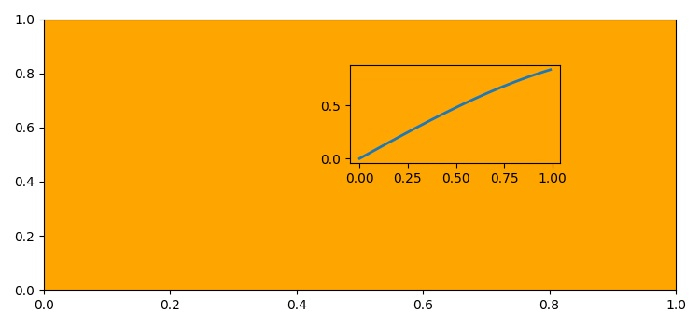# How to make axes transparent in Matplotlib?

To make axes transparent in matplotlib, we can take the following steps,

• Set the figure size and adjust the padding between and around the subplots.

• Create a new figure or activate an existing figure using figure() method.

• Add an '~.axes.Axes' to the figure as part of a subplot arrangement.

• Set face color of the current axes.

• Add an axes to the figure.

• Create t and s data using numpy.

• Plot t and s data points using plot() method on axis 2 (from step 5).

• To make the axis transparent, use set_alpha() method and keep alpha value minimum.

• To display the figure, use show() method.

## Example

import matplotlib.pyplot as plt
import numpy as np

plt.rcParams["figure.figsize"] = [7.50, 3.50]
plt.rcParams["figure.autolayout"] = True

fig = plt.figure()

ax1.set_facecolor('orange')
ax2 = fig.add_axes([0.5, 0.5, 0.3, 0.3])

t = np.arange(0, 1, 0.01)
s = np.sin(t)

ax2.plot(t, s, linewidth=2)
ax2.patch.set_alpha(0.01)

plt.show()

## Output